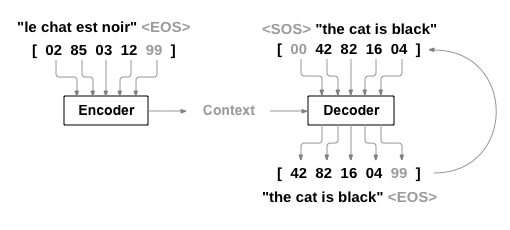# Recurrent decoder's input in an auto-encoder with batch training

I’m creating a sequence to sequence model based on an auto-encoder to map a sequence of words to another sequence of words. The data I am using is sizable so batch training is essential. Now, defining the encoder seems to be straightforward. But I was wondering what should be the input of the decoder since at each time step `t` the decoder receives a hidden vector and its own output from `t-1` as input.

This can be seen in the following figure from Sean Robertson’s pytorch tutorial’s and in Sutskever et al (2014).I am not sure how to pass the output of the decoder to itself as an input of the next time step when training with batch.
Below is how I define an encoder and decoder, but the input of the decoder is missing.

``````class seq2seq(nn.Module):

def __init__(self, ):
super(seq2seq, self).__init__()

self.embed = nn.Embedding(embedding_input_size, embedding_hidden_size)

self.encoder = nn.GRU(input_size=embedding_hidden_size,
hidden_size=encoder_hidden_size,
num_layers=1)

self.decoder = nn.GRU(input_size=encoder_hidden_size,
hidden_size=decoder_hidden_size,
num_layers=1)

# sos_vec is the one hot vector representing the start of sequence
decoder_first_inp = Variable(torch.LongTensor(sos_vec))

def forward(self, input_btch, hidden_btch):

encO, encH = self.encoder(input_btch, hidden_btch)

decO, decH = self.decoder(???, encH)``````

The inputs of your decoder must be the context (derivated from the encoder’s final hidden) that is used to initialize the hidden for your decoder, and the target sequence (sos+“the cat is black”). So you should have something lie this (I’m trying to use your notations):

``````
def forward(self, input_btch, hidden_btch, target_btch):
encO, encH = self.encoder(input_btch, hidden_btch)
context = f(encH)
decH = context
decI = torch.cat(sos, target_btch)
decO, _ = self.decoder(decI, decH)
return decO
``````
2 Likes

What do you mean by f()? As I understand we should directly put `encH` into decoder

Yes, you can directly put encH.

Just in case you want to have different hidden sizes for encoder and decoder you can add a linear layer to change the size of encH or, also, sometimes the context is sampled from a distribution derived from encH: https://arxiv.org/pdf/1511.06349.pdf, especially if the space is continuous

2 Likes

I see, thanks for the explanation.

How can I run this on multiple GPUs?

You can use `torch.multiprocessing`: http://pytorch.org/docs/master/notes/multiprocessing.html
You can find a clean example of a single model updated by independent workers on different GPUs here: https://github.com/ikostrikov/pytorch-a3c, it’s about reinforcement learning, but I think you should easily adapt it to train your RNN

@alexis-jacq This works for training, any thoughts on how to use the trained decoder for predicting a sequence?

Simply load the trained shared model in a test function, that you throw as a new thread in your `main.py` after stoping the training threads:

``````def test(args, shared_model):
Thanks, that’s sounds like a good idea. As an alternative, I made my decoder accept the Boolean input argument `predict`. When this argument is true, the input of each time step becomes the output of the previous time step. I think this is more memory efficient, given that I am running these models on a GPU. But then again, I assume the weights do not consume so much memory unless the model has many layers.